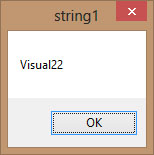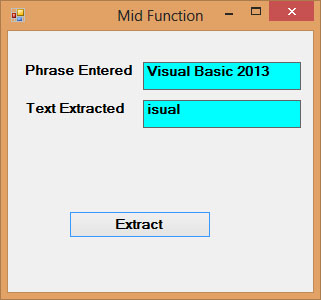# Visual Basic 2013 Lesson 12: Strings Manipulation

#### [Lesson 11] << [Contents] >> [Lesson 13]

String manipulation means writing code to process non-numeric variables. Examples of the string are names, addresses, gender, cities, book titles, alphanumeric characters (@,#,\$,%,^,&,*, etc) and more. In Visual Basic 2013, a string is a single unit of data that made up of a series of characters.They include letters, digits, alphanumeric symbols and more. It is treated as the String data type and cannot be manipulated mathematically though it might consist of numbers.

### 12.1 String Manipulation Using + and & signs.

In Visual Basic 2013, strings can be manipulated using the & sign and the + sign, both perform the string concatenation. It means combining two or more smaller strings into larger strings. For example, we can join “Visual”,”Basic” and “2013″ into “Visual Basic 2013″ using “Visual”&”Basic” or “Visual “+”Basic”, as shown in the Examples below:

#### Example 12.1

```Private Sub Button1_Click(ByVal sender As System.Object, ByVal e As System.EventArgs) Handles Button1.ClickDim
text1, text2, text3, text4 As String
text1 = “Visual”
text2 = “Basic”
text3=”2013″
text4 = text1 + text2+text3
MsgBox (text4)
End Sub
```

The line text4=text1+ text2 + text3 can be replaced by text4=text1 & text2 &text3 and produces the same output. However, if one of the variables is declared as numeric data type, you cannot use the + sign, you can only use the & sign.

The output is shown in Figure 12.1:

#### Example 12.2

```Private Sub Button1_Click(ByVal sender As System.Object, ByVal e As System.EventArgs) Handles Button1.Click
Dim text1, text3 as string
Dim Text2 As Integer
text1 = “Visual”
text2=22
text3=text1+text2
MsgBox(text3)
End Sub
```

This code will produce an error because of data mismatch. The error message appears as follows:

However, using & instead of + will be all right.

```Dim text1, text3 as string
Dim Text2 As Integer
text1 = “Visual”
text2=22
text3=text1 & text2
MsgBox(text3)
```Figure 12.3

### 12.2 String Manipulation Using VB2013 Built-in Functions

A function is similar to a normal procedure but the main purpose of the function is to accept a certain input and return a value which is passed on to the main program to finish the execution.There are numerous string manipulation functions that are built into Visual Basic 2013.

#### 12.2 (a) The Len Function

The Len function returns an integer value which is the length of a phrase or a sentence, including the empty spaces. The syntax is

`Len (“Phrase”)`

For example,

```Len (Visual Basic) = 12 and Len (“welcome to VB tutorial”) = 22
```

#### Example 12.3

```Private Sub Button1_Click(ByVal sender As System.Object, ByVal e As System.EventArgs) Handles Button1.Click
Dim MyText as String
MyText="Visual Basic 2013"
MsgBox(Len(MyText))
End Sub
```

The output:Figure 12.4

#### 12.2(b) The Right Function

The Right function extracts the right portion of a phrase. The syntax for Visual Basic 6 is

`Right (“Phrase”, n)`

Where n is the starting position from the right of the phase where the portion of the phrase is going to be extracted. For example,

```Right(“Visual Basic”, 4) = asic
```

However, this syntax is not applicable in Visual Basic 2013. In VB2013, we need to use the following syntax

`Microsoft.VisualBasic.Right(“Phrase”,n)`

The reason of using the full reference is because many objects have the Right properties so using Right on its own will make it ambiguous to Visual Basic 2013.

#### Example 12.4

```Private Sub Button1_Click(ByVal sender As System.Object, ByVal e As System.EventArgs) Handles Button1.Click
Dim MyText As String
MyText="Visual Basic"
MsgBox(Microsoft.VisualBasic.Right(MyText, 4))
End Sub
```

The above program returns four rightmost characters of the phrase entered into the textbox.

The Output:

#### 12.2(c)The Left Function

The Left function extracts the left portion of a phrase. The syntax  is

`Microsoft.VisualBasic.Left(“Phrase”,n)`

Where n is the starting position from the left of the phase where the portion of the phrase is will be extracted. For example,

```Microsoft.VisualBasic.Left (“Visual Basic”, 4) = Visu .
```

#### 12.2 (d) The Mid Function

The Mid function is used to retrieve a part of the text from a given phrase. The syntax of the Mid Function is

`Mid(phrase, position,n)`

where

phrase is the string from which a part of the text is to be retrieved.

position is the starting position of the phrase from which the retrieving process begins.

n is the number of characters to retrieve.

Example 12.5:

```Private Sub Button1_Click(sender As Object, e As EventArgs) Handles Button1.Click
Dim myPhrase As String
LblPhrase.Text = myPhrase
LblExtract.Text = Mid(myPhrase, 2, 6)
End Sub
```

* In this example, when the user clicks the button, an input box will pop up prompting the user to enter a phrase. After a phrase is entered and the OK button is pressed, the label will show the extracted text starting from position 2 of the phrase and the number of characters extracted is 6.Figure 12.6

#### 12.2(e) The Trim Function

The Trim function trims the empty spaces on both sides of the phrase. The syntax is

`Trim(“Phrase”)`

.For example,

`Trim (” Visual Basic “) = Visual basic`

Example 13.4

```Private Sub Button1_Click(ByVal sender As System.Object, ByVal e As System.EventArgs) Handles Button1.Click
Dim myPhrase As String
Label1.Text = Trim(myPhrase)
End Sub
```

#### 12.2(f) The Ltrim Function

The Ltrim function trims the empty spaces of the left portion of the phrase. The syntax is

`Ltrim(“Phrase”)`

.For example,

```Ltrim ("    Visual Basic 2013")= Visual basic 2013
```

#### 12.2(g)The Rtrim Function

The Rtrim function trims the empty spaces of the right portion of the phrase. The syntax is

`Rtrim(“Phrase”)`

.For example,

`Rtrim (“Visual Basic 2013     “) = Visual Basic 2013`

#### 12.2(h) The InStr function

The InStr function looks for a phrase that is embedded within the original phrase and returns the starting position of the embedded phrase. The syntax  is

`Instr (n, original phase, embedded phrase)`

Where n is the position where the Instr function will begin to look for the embedded phrase. For example

`Instr(1, “Visual Basic 2013 “,”Basic”)=8`

*The function returns a numeric value.

You can write a program code as shown below:

```Private Sub Button1_Click(ByVal sender As System.Object, ByVal e As System.EventArgs) Handles Button1.Click
Label1.Text = InStr(1, “Visual Basic”, “Basic”)
End Sub
```

#### 12.2(i) The Ucase and the Lcase Functions

The Ucase function converts all the characters of a string to capital letters. On the other hand, the Lcase function converts all the characters of a string to small letters.

The syntaxes  are

```Microsoft.VisualBasic.UCase(Phrase)

Microsoft.VisualBasic.LCase(Phrase)
```

For example,

```Microsoft.VisualBasic.Ucase(“Visual Basic”) =VISUAL BASIC

Microsoft.VisualBasic.Lcase(“Visual Basic”) =visual basic
```

#### 12.2(j)The Chr and the Asc functions

The Chr function returns the string that corresponds to an ASCII code while the Asc function converts an ASCII character or symbol to the corresponding ASCII code. ASCII stands for “American Standard Code for Information Interchange”. Altogether there are 255 ASCII codes and as many ASCII characters. Some of the characters may not be displayed as they may represent some actions such as the pressing of a key or produce a beep sound. The syntax of the Chr function is

```Chr(charcode)
```

and the format of the Asc function is

```Asc(Character)
```

The following are some examples:

```Chr(65)=A, Chr(122)=z, Chr(37)=% ,

Asc(“B”)=66, Asc(“&”)=38
```